# GRAVITATION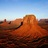Associate Professor at Erode sengunthar engineering college à Erode sengunthar engineering college
4 Dec 2022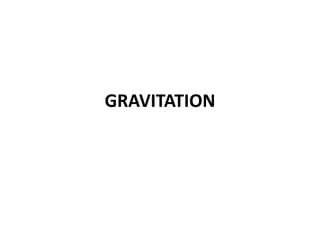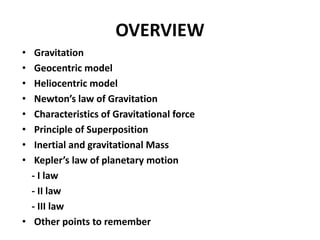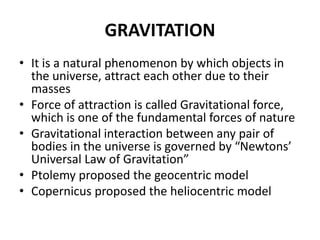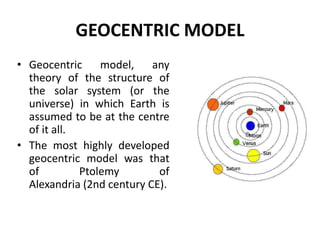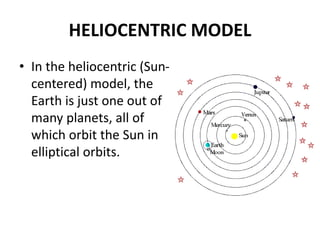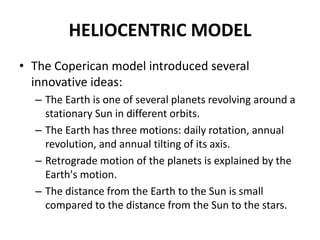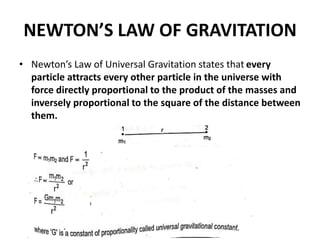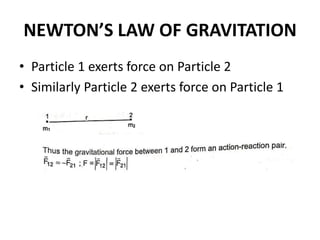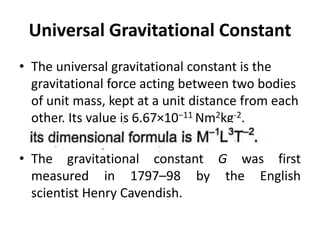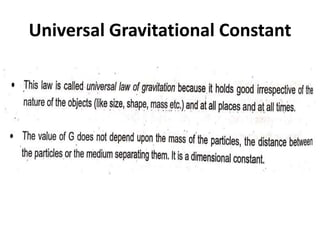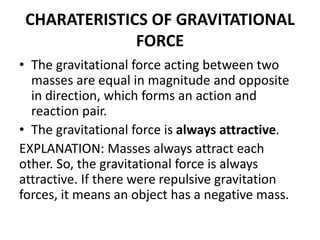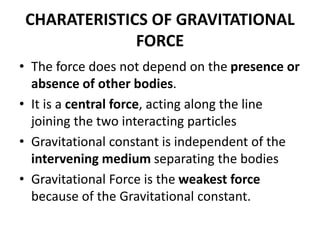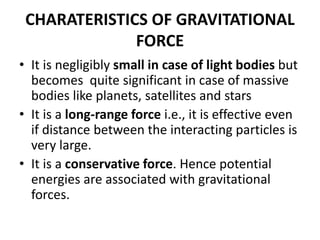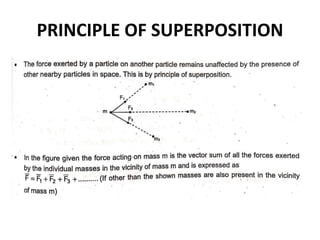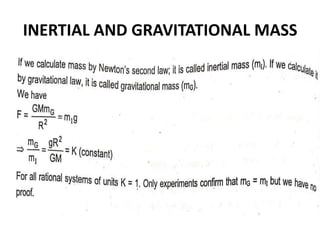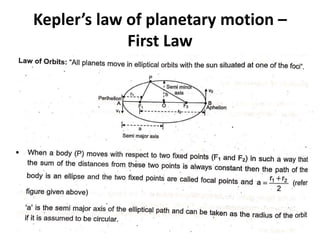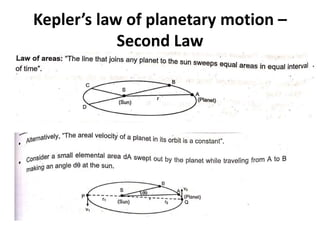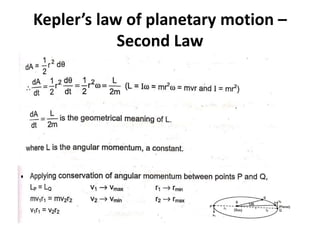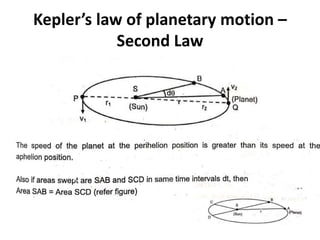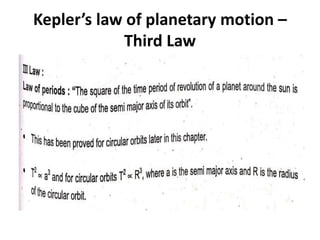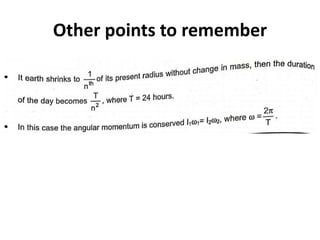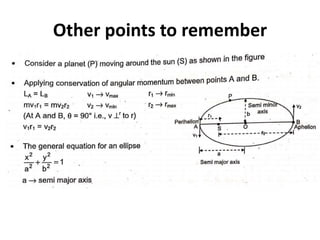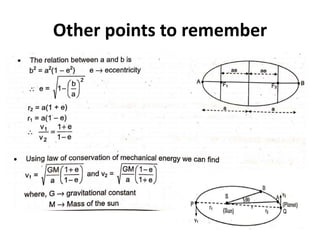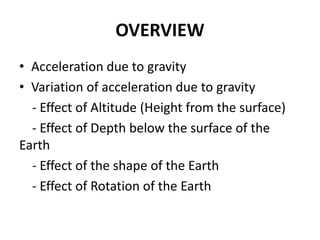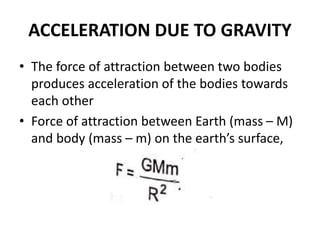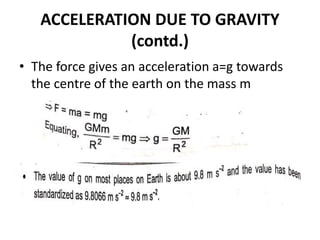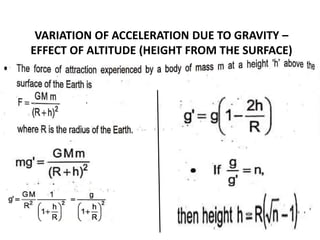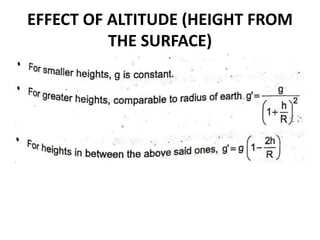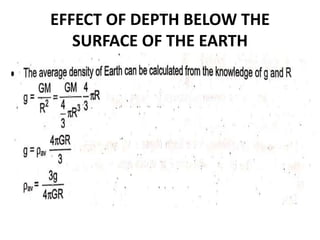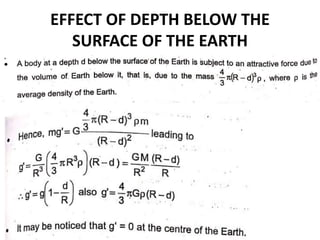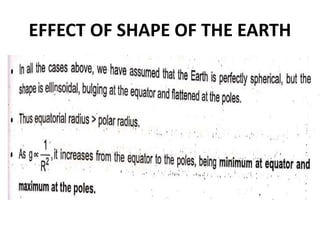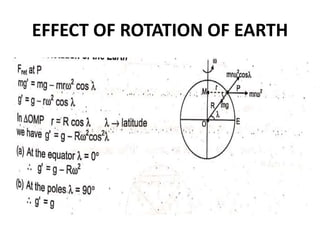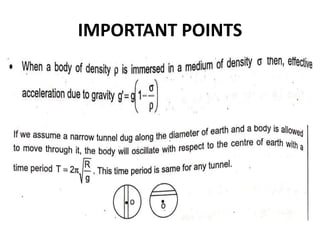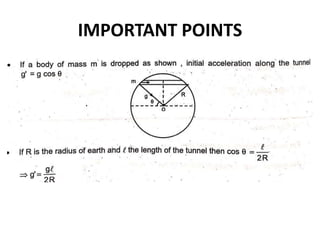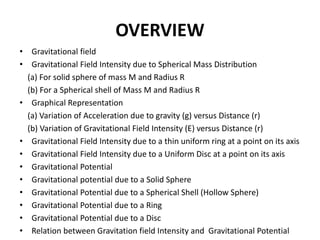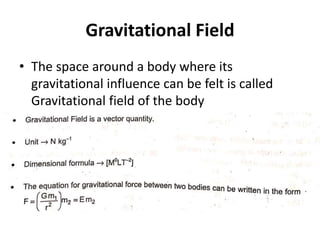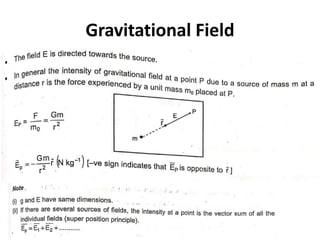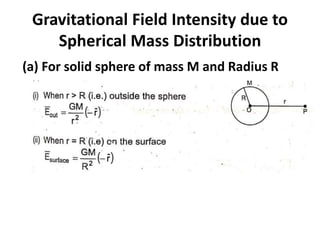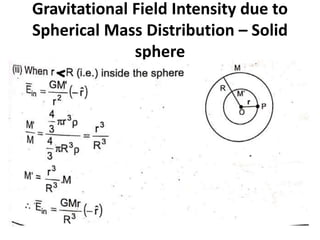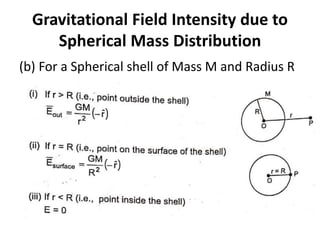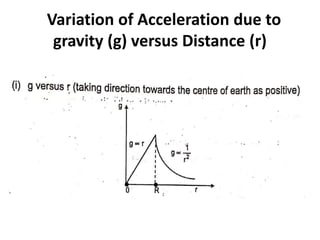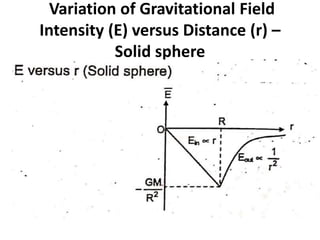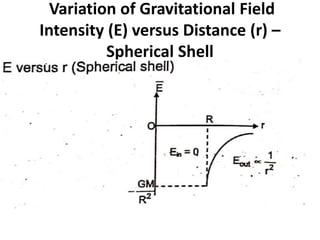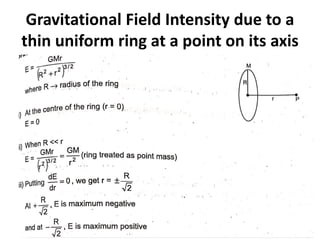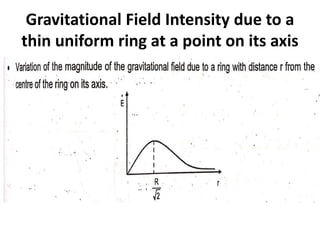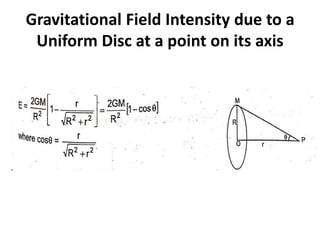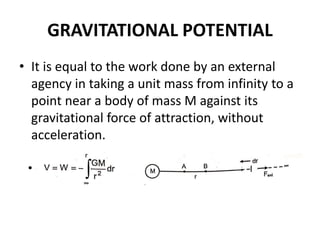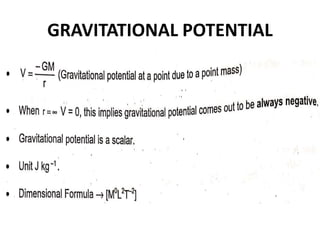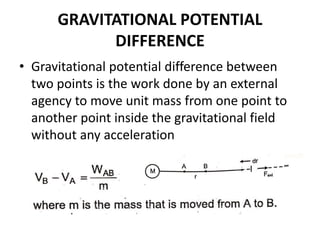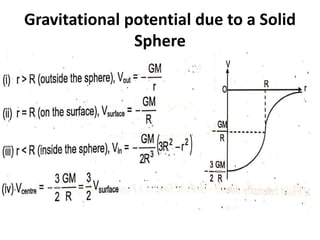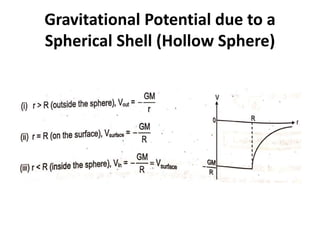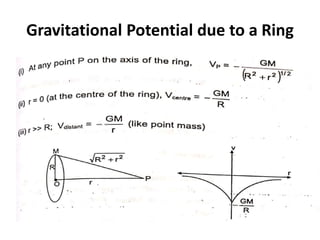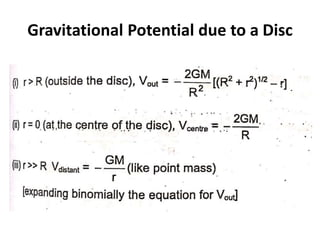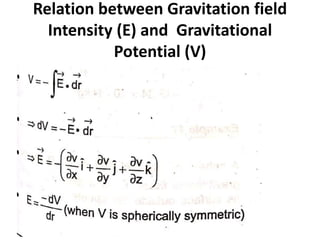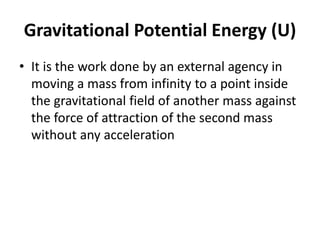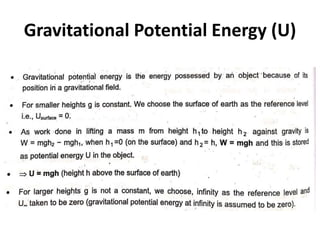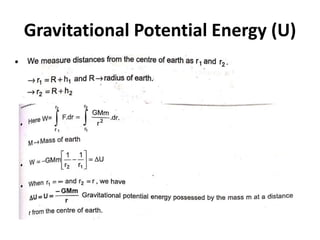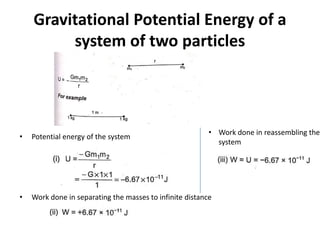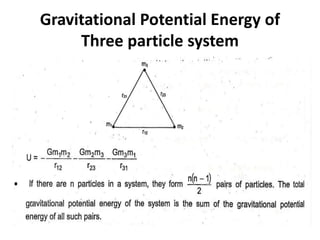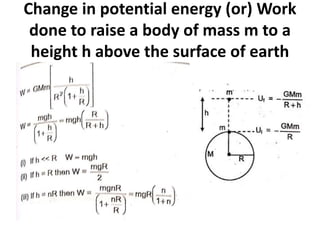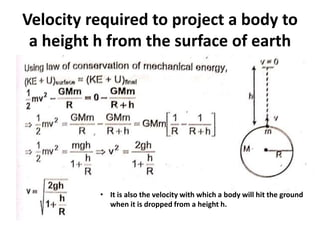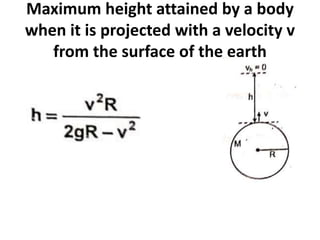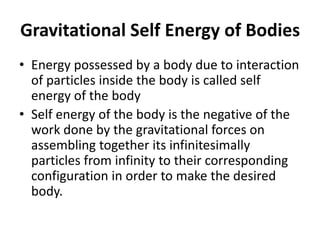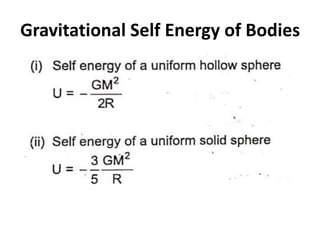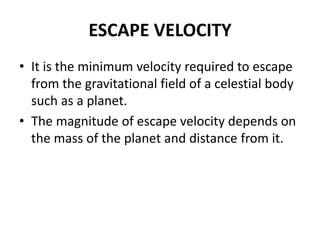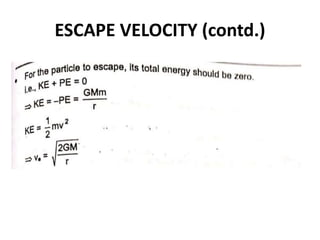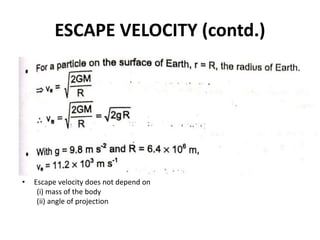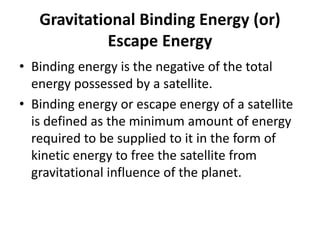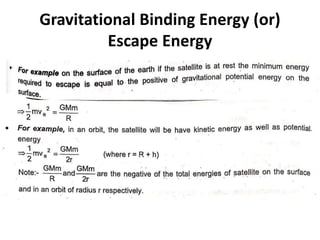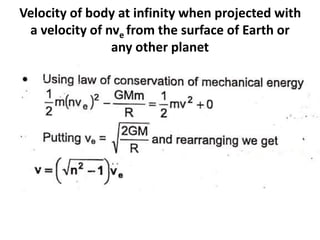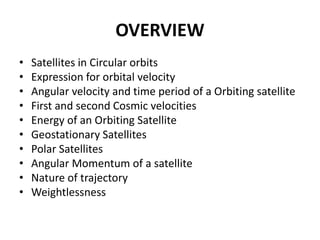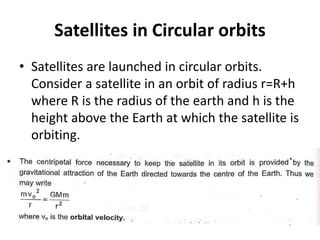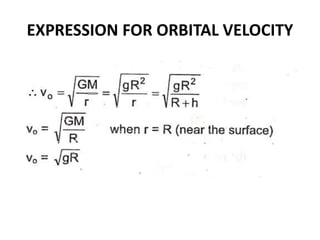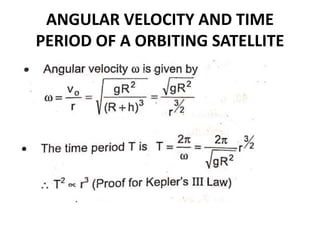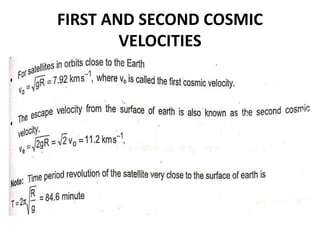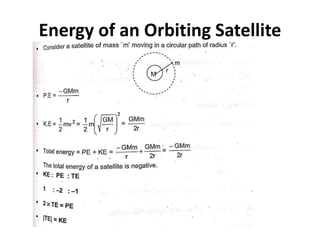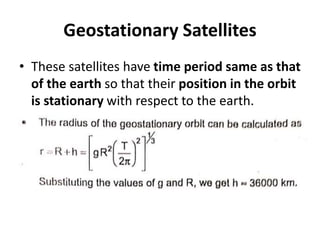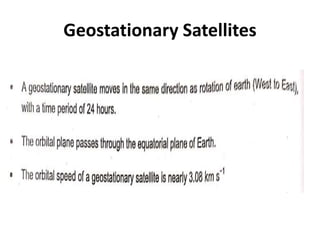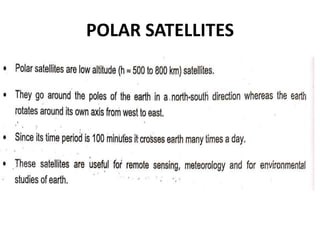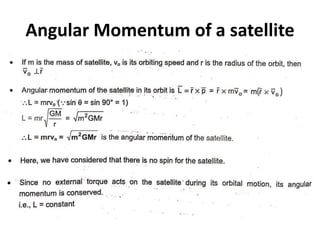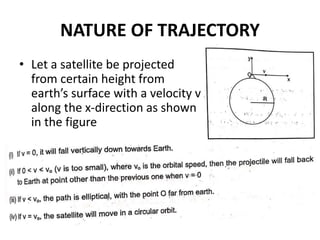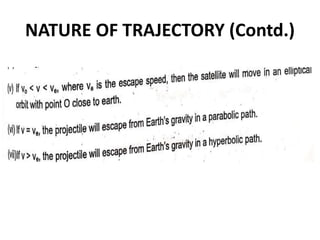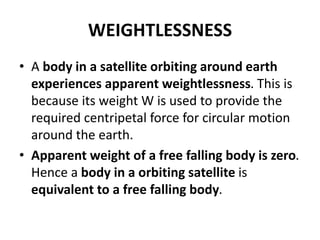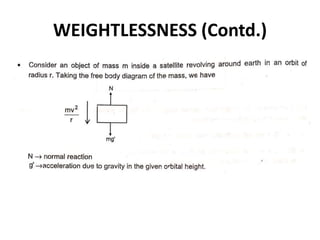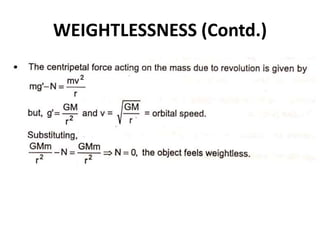1 sur 92

### GRAVITATION

• 3. OVERVIEW • Gravitation • Geocentric model • Heliocentric model • Newton’s law of Gravitation • Characteristics of Gravitational force • Principle of Superposition • Inertial and gravitational Mass • Kepler’s law of planetary motion - I law - II law - III law • Other points to remember
• 4. GRAVITATION • It is a natural phenomenon by which objects in the universe, attract each other due to their masses • Force of attraction is called Gravitational force, which is one of the fundamental forces of nature • Gravitational interaction between any pair of bodies in the universe is governed by “Newtons’ Universal Law of Gravitation” • Ptolemy proposed the geocentric model • Copernicus proposed the heliocentric model
• 5. GEOCENTRIC MODEL • Geocentric model, any theory of the structure of the solar system (or the universe) in which Earth is assumed to be at the centre of it all. • The most highly developed geocentric model was that of Ptolemy of Alexandria (2nd century CE).
• 6. HELIOCENTRIC MODEL • In the heliocentric (Sun- centered) model, the Earth is just one out of many planets, all of which orbit the Sun in elliptical orbits.
• 7. HELIOCENTRIC MODEL • The Coperican model introduced several innovative ideas: – The Earth is one of several planets revolving around a stationary Sun in different orbits. – The Earth has three motions: daily rotation, annual revolution, and annual tilting of its axis. – Retrograde motion of the planets is explained by the Earth's motion. – The distance from the Earth to the Sun is small compared to the distance from the Sun to the stars.
• 8. NEWTON’S LAW OF GRAVITATION • Newton’s Law of Universal Gravitation states that every particle attracts every other particle in the universe with force directly proportional to the product of the masses and inversely proportional to the square of the distance between them.
• 9. NEWTON’S LAW OF GRAVITATION • Particle 1 exerts force on Particle 2 • Similarly Particle 2 exerts force on Particle 1
• 10. Universal Gravitational Constant • The universal gravitational constant is the gravitational force acting between two bodies of unit mass, kept at a unit distance from each other. Its value is 6.67×10−11 Nm2kg-2. • The gravitational constant G was first measured in 1797–98 by the English scientist Henry Cavendish.
• 12. CHARATERISTICS OF GRAVITATIONAL FORCE • The gravitational force acting between two masses are equal in magnitude and opposite in direction, which forms an action and reaction pair. • The gravitational force is always attractive. EXPLANATION: Masses always attract each other. So, the gravitational force is always attractive. If there were repulsive gravitation forces, it means an object has a negative mass.
• 13. CHARATERISTICS OF GRAVITATIONAL FORCE • The force does not depend on the presence or absence of other bodies. • It is a central force, acting along the line joining the two interacting particles • Gravitational constant is independent of the intervening medium separating the bodies • Gravitational Force is the weakest force because of the Gravitational constant.
• 14. CHARATERISTICS OF GRAVITATIONAL FORCE • It is negligibly small in case of light bodies but becomes quite significant in case of massive bodies like planets, satellites and stars • It is a long-range force i.e., it is effective even if distance between the interacting particles is very large. • It is a conservative force. Hence potential energies are associated with gravitational forces.
• 17. Kepler’s law of planetary motion – First Law
• 18. Kepler’s law of planetary motion – Second Law
• 19. Kepler’s law of planetary motion – Second Law
• 20. Kepler’s law of planetary motion – Second Law
• 21. Kepler’s law of planetary motion – Third Law
• 22. Other points to remember
• 23. Other points to remember
• 24. Other points to remember
• 26. OVERVIEW • Acceleration due to gravity • Variation of acceleration due to gravity - Effect of Altitude (Height from the surface) - Effect of Depth below the surface of the Earth - Effect of the shape of the Earth - Effect of Rotation of the Earth
• 27. ACCELERATION DUE TO GRAVITY • The force of attraction between two bodies produces acceleration of the bodies towards each other • Force of attraction between Earth (mass – M) and body (mass – m) on the earth’s surface,
• 28. ACCELERATION DUE TO GRAVITY (contd.) • The force gives an acceleration a=g towards the centre of the earth on the mass m
• 29. VARIATION OF ACCELERATION DUE TO GRAVITY – EFFECT OF ALTITUDE (HEIGHT FROM THE SURFACE)
• 30. EFFECT OF ALTITUDE (HEIGHT FROM THE SURFACE)
• 31. EFFECT OF DEPTH BELOW THE SURFACE OF THE EARTH
• 32. EFFECT OF DEPTH BELOW THE SURFACE OF THE EARTH
• 33. EFFECT OF SHAPE OF THE EARTH
• 34. EFFECT OF ROTATION OF EARTH
• 38. OVERVIEW • Gravitational field • Gravitational Field Intensity due to Spherical Mass Distribution (a) For solid sphere of mass M and Radius R (b) For a Spherical shell of Mass M and Radius R • Graphical Representation (a) Variation of Acceleration due to gravity (g) versus Distance (r) (b) Variation of Gravitational Field Intensity (E) versus Distance (r) • Gravitational Field Intensity due to a thin uniform ring at a point on its axis • Gravitational Field Intensity due to a Uniform Disc at a point on its axis • Gravitational Potential • Gravitational potential due to a Solid Sphere • Gravitational Potential due to a Spherical Shell (Hollow Sphere) • Gravitational Potential due to a Ring • Gravitational Potential due to a Disc • Relation between Gravitation field Intensity and Gravitational Potential
• 39. Gravitational Field • The space around a body where its gravitational influence can be felt is called Gravitational field of the body
• 41. Gravitational Field Intensity due to Spherical Mass Distribution (a) For solid sphere of mass M and Radius R
• 42. Gravitational Field Intensity due to Spherical Mass Distribution – Solid sphere
• 43. Gravitational Field Intensity due to Spherical Mass Distribution (b) For a Spherical shell of Mass M and Radius R
• 44. Variation of Acceleration due to gravity (g) versus Distance (r)
• 45. Variation of Gravitational Field Intensity (E) versus Distance (r) – Solid sphere
• 46. Variation of Gravitational Field Intensity (E) versus Distance (r) – Spherical Shell
• 47. Gravitational Field Intensity due to a thin uniform ring at a point on its axis
• 48. Gravitational Field Intensity due to a thin uniform ring at a point on its axis
• 49. Gravitational Field Intensity due to a Uniform Disc at a point on its axis
• 50. GRAVITATIONAL POTENTIAL • It is equal to the work done by an external agency in taking a unit mass from infinity to a point near a body of mass M against its gravitational force of attraction, without acceleration.
• 52. GRAVITATIONAL POTENTIAL DIFFERENCE • Gravitational potential difference between two points is the work done by an external agency to move unit mass from one point to another point inside the gravitational field without any acceleration
• 53. Gravitational potential due to a Solid Sphere
• 54. Gravitational Potential due to a Spherical Shell (Hollow Sphere)
• 57. Relation between Gravitation field Intensity (E) and Gravitational Potential (V)
• 59. OVERVIEW • Gravitational Potential Energy (U) • Gravitational Potential Energy of a system of two particles • Gravitational Potential Energy of three particle system • Change in potential energy (or) Work done to raise a body of mass m to a height h above the surface of earth • Velocity required to project a body to a height h from the surface of earth • Maximum height attained by a body when it is projected with a velocity v from the surface of the earth • ‘Gravitational self energy’ of bodies • Escape velocity • Gravitational Binding energy (or) Escape Energy • Velocity of body at infinity when projected with a velocity of nve from the surface of Earth or any other planet
• 60. Gravitational Potential Energy (U) • It is the work done by an external agency in moving a mass from infinity to a point inside the gravitational field of another mass against the force of attraction of the second mass without any acceleration
• 63. Gravitational Potential Energy of a system of two particles • Potential energy of the system • Work done in separating the masses to infinite distance • Work done in reassembling the system
• 64. Gravitational Potential Energy of Three particle system
• 65. Change in potential energy (or) Work done to raise a body of mass m to a height h above the surface of earth
• 66. Change in potential energy (or) Work done to raise a body of mass m to a height h above the surface of earth
• 67. Velocity required to project a body to a height h from the surface of earth • It is also the velocity with which a body will hit the ground when it is dropped from a height h.
• 68. Maximum height attained by a body when it is projected with a velocity v from the surface of the earth
• 69. Gravitational Self Energy of Bodies • Energy possessed by a body due to interaction of particles inside the body is called self energy of the body • Self energy of the body is the negative of the work done by the gravitational forces on assembling together its infinitesimally particles from infinity to their corresponding configuration in order to make the desired body.
• 71. ESCAPE VELOCITY • It is the minimum velocity required to escape from the gravitational field of a celestial body such as a planet. • The magnitude of escape velocity depends on the mass of the planet and distance from it.
• 73. ESCAPE VELOCITY (contd.) • Escape velocity does not depend on (i) mass of the body (ii) angle of projection
• 74. Gravitational Binding Energy (or) Escape Energy • Binding energy is the negative of the total energy possessed by a satellite. • Binding energy or escape energy of a satellite is defined as the minimum amount of energy required to be supplied to it in the form of kinetic energy to free the satellite from gravitational influence of the planet.
• 75. Gravitational Binding Energy (or) Escape Energy
• 76. Velocity of body at infinity when projected with a velocity of nve from the surface of Earth or any other planet
• 78. OVERVIEW • Satellites in Circular orbits • Expression for orbital velocity • Angular velocity and time period of a Orbiting satellite • First and second Cosmic velocities • Energy of an Orbiting Satellite • Geostationary Satellites • Polar Satellites • Angular Momentum of a satellite • Nature of trajectory • Weightlessness
• 79. Satellites in Circular orbits • Satellites are launched in circular orbits. Consider a satellite in an orbit of radius r=R+h where R is the radius of the earth and h is the height above the Earth at which the satellite is orbiting.
• 81. ANGULAR VELOCITY AND TIME PERIOD OF A ORBITING SATELLITE
• 82. FIRST AND SECOND COSMIC VELOCITIES
• 83. Energy of an Orbiting Satellite
• 84. Geostationary Satellites • These satellites have time period same as that of the earth so that their position in the orbit is stationary with respect to the earth.
• 87. Angular Momentum of a satellite
• 88. NATURE OF TRAJECTORY • Let a satellite be projected from certain height from earth’s surface with a velocity v along the x-direction as shown in the figure
• 90. WEIGHTLESSNESS • A body in a satellite orbiting around earth experiences apparent weightlessness. This is because its weight W is used to provide the required centripetal force for circular motion around the earth. • Apparent weight of a free falling body is zero. Hence a body in a orbiting satellite is equivalent to a free falling body.